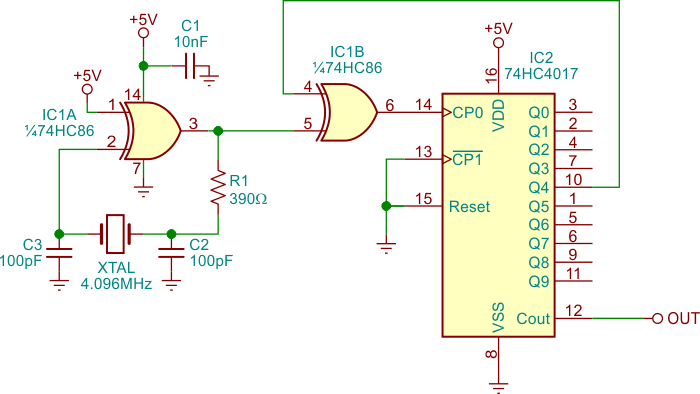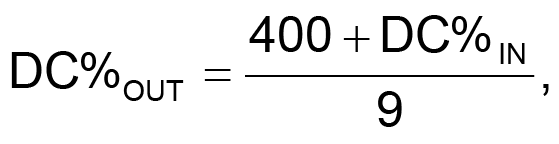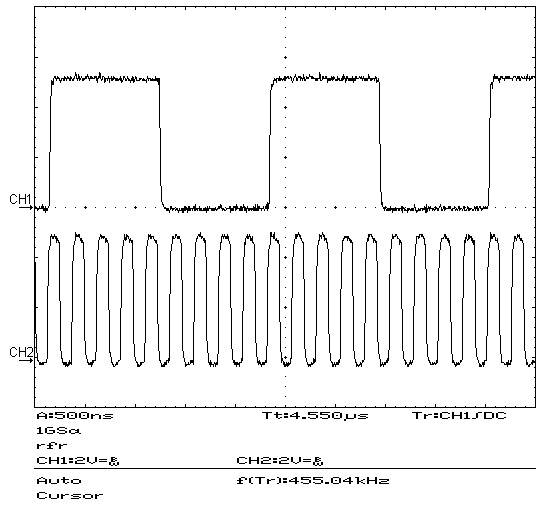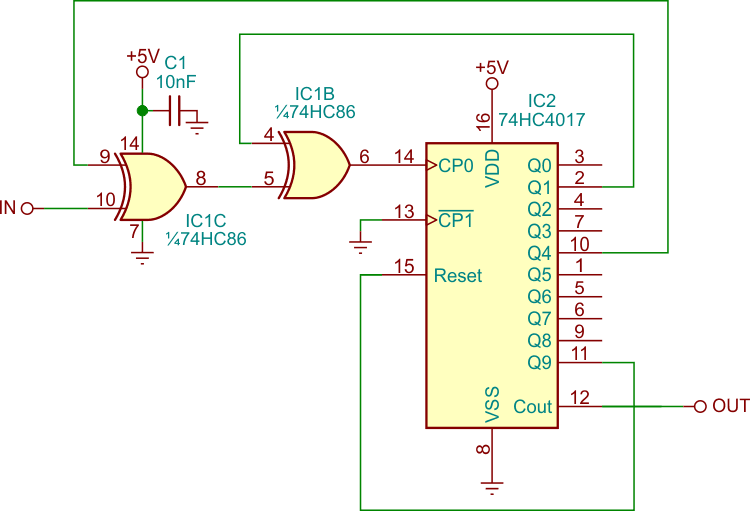# Divider generates accurate 455kHz square-wave signal

## Texas Instruments CD74HC4017 CD74HC86

The circuit here is an oscillator with an unusual frequency divider that I designed when I was developing an RF system. I needed a stable and accurate 455 kHz digital signal generator that used as few components as possible. The signal generator also had to have a duty cycle of, or very close to, 50% to nullify or minimize the second harmonic content.

I first looked at oscillators based on 455 kHz ceramic resonators as well as on 455 kHz ceramic filters. When testing, however, I obtained signals of 448 kHz and 462 kHz, which lacked the accuracy I needed. But I had on hand a 4.096 MHz quartz crystal. By making it oscillate in a stable way at approximately 4.095 MHz and dividing that frequency by nine with a 50% duty cycle output, I obtained the result I was looking for.

The design, shown in Figure 1, uses two integrated circuits and some capacitors and resistors, besides the crystal. It implements a Pierce-type oscillator with an XOR gate (IC1) as the active element. An XOR gate working as an inverter is not the most common gate used for this type of oscillator, but there were three of them left unused elsewhere in my system so I gave them a try. The oscillator worked reliably and accurately in that configuration.Figure 1. This design uses a Pierce oscillator based on an XOR gate and generates 455 kHz with 50% duty cycle.

The crystal used was a parallel type for a 20 pF load. I obtained 4.0954 MHz by using 100 pF for C2 and C3. That frequency divided by 9 gives 455.04 kHz, a value that was accurate enough for my design’s needs.

The second IC (IC2) is a 74HC4017, a Johnson counter of which I used the Carry Out line (pin 12) as the circuit output and which I configured to be triggered by rising edges. With the Reset input (pin 15) held at logic level 0, the Carry Out line (pin 12) takes level 1 when the counter’s state is between zero and four, and level 0 if its state is between five and nine.

You might think that an option for this circuit would be to connect Q9 (pin 11) to the Reset input. In that case, however, while the frequency output would have been 4.0954 MHz divided by 9, the output duty cycle would have been 55.56% (or 44.44% if inverting the output). That would not have satisfied the need to reduce or eliminate the output’s second harmonic.

Searching for a duty cycle closer to 50%, I added XOR gate IC1B connected to the counter’s Q4 (pin 10) output. With that change, the counter will now trigger on the input’s rising edges while Q4 is level 0, and on the falling edges when Q4 is level 1. As a consequence, the circuit outputs a level 1 for four clock pulses plus the time in the fifth pulse that the input is at level 1. The output goes to level 0 when the fifth pulse goes to level 0, where it stays for the following four pulses. Thus, the input’s frequency gets divided by nine and, if the input duty cycle is 50%, the output will also be.

If the input frequency is not quite 50%, the output will also be off, but not by as much. The input and output duty cycles are related by the formula:where DC%out and DC%in are the output and input duty cycles respectively, expressed as percentages between 0% and 100%. So, if DC%IN falls between 0% and 100%, the output duty cycle will be between 44.44% and 55.56%, no worse than the case I mentioned above.

Tests of the circuit produced the oscilloscope capture shown in Figure 2. Both the 4.0954 MHz oscillator signal (at the bottom) and the 455.04 kHz output signal (at the top) have a duty cycle of 50%.Figure 2. Test results show that both the input signal and the divide-by-nine output have 50% duty cycles.

It is possible to make a divide-by-seven circuit by using another XOR gate, as shown in Figure 3. This will also produce a 50%-duty-cycle output if the duty cycle input is 50%.Figure 3. Adding another XOR gate allows the circuit to provide a divide-by-seven with 50% duty cycle output.

I have tested this circuit with a variety of crystals and it worked properly.

## Materials on the topic

EDN